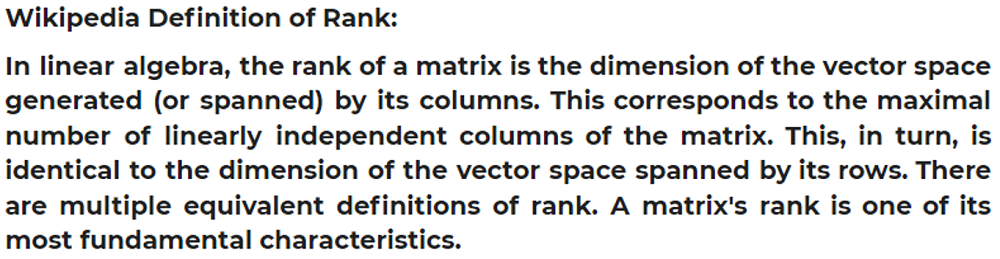# Python | Rank of a Matrix

Rank of a Matrix in Python: Here, we are going to learn about the Rank of a Matrix and how to find it using Python code?
Submitted by Anuj Singh, on July 17, 2020

The rank of a Matrix is defined as the number of linearly independent columns present in a matrix. The number of linearly independent columns is always equal to the number of linearly independent rows. In this article, we are going to find Rank of a Matrix.There is an inbuilt function defined in numpy.linalg package as shown below,

```rank = numpy.linalg.matrix_rank(a)
```

## Python code to find rank of a matrix

```# Linear Algebra Learning Sequence
# Rank of a Matrix

import numpy as np

a = np.array([[4,5,8], [7,1,4], [5,5,5], [2,3,6]])

rank = np.linalg.matrix_rank(a)

print('Matrix : ', a)
print('Rank of the given Matrix : ',rank)
```

Output:

```Matrix :  [[4 5 8]
[7 1 4]
[5 5 5]
[2 3 6]]
Rank of the given Matrix :  3
```

Preparation

What's New

Top Interview Coding Problems/Challenges!